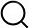# Also in the Article

2.6. Radiological model building and validation

Procedure

The Radscore of each sequence was considered a variable. A radiological combined model was built based on Radscores of four sequences using multiple logistic regression. To estimate the prediction error and confidence interval, we further tested the proposed model using a 1000‐iteration bootstrap analysis on the overall dataset. The performance of the radiological model of four sequences was validated by a ROC curve. The radiological combined model was validated by a ROC curve, nomogram, calibration, and DCA.

Q&A